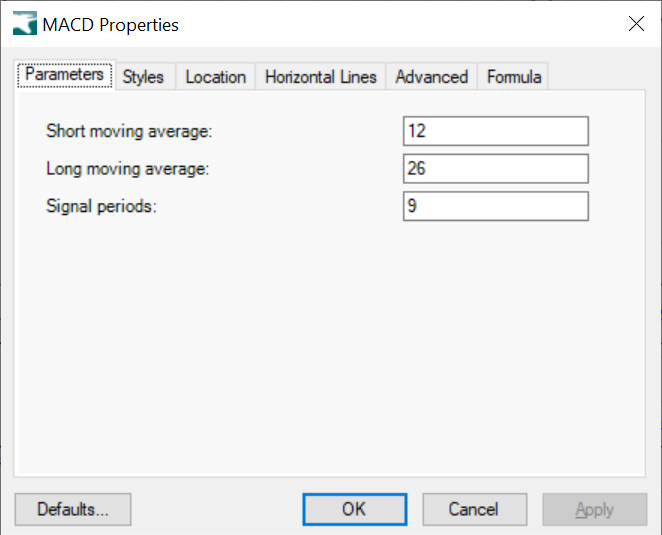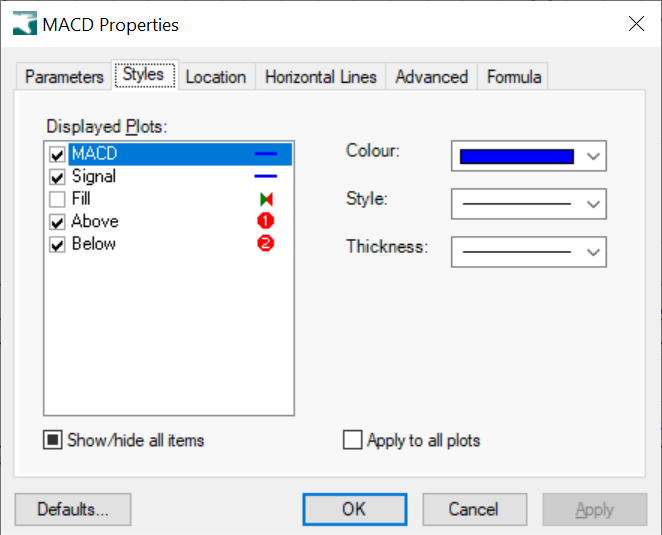# MACD

## Moving Average Convergence Divergence

The MACD is calculated by subtracting the 26-period Exponential Moving Average (EMA) from the 12-period EMA. The result of that calculation is the MACD line. A nine-day EMA of the MACD called the signal line, is then plotted on top of the MACD line, which can function as a trigger for buy and sell signals. Source: MACD